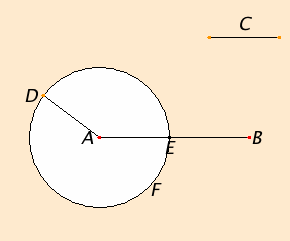# Proposition 3

To cut off from the greater of two given unequal straight lines a straight line equal to the less.

Let AB and C be the two given unequal straight lines, and let AB be the greater of them.It is required to cut off from AB the greater a straight line equal to C the less.

Place AD at the point A equal to the straight line C, and describe the circle DEF with center A and radius AD.

I.Def.15

Now, since the point A is the center of the circle DEF, therefore AE equals AD.

C.N.1

But C also equals AD, therefore each of the straight lines AE and C equals AD, so that AE also equals C.

Therefore, given the two straight lines AB and C, AE has been cut off from AB the greater equal to C the less.

Q.E.F.

## Guide

Now it is clear that the purpose of Proposition 2 is to effect the construction in this proposition.

According to Proclus (410–485 C.E.) in his Commentary on Book I, Hippocrates of Chios (fl. ca. 430 B.C.E.) was the first to write an Elements. Leon and Theudius also wrote versions before Euclid (fl. ca. 295 B.C.E.). These other Elements have all been lost since Euclid’s replaced them. It is conceivable that in some of these earlier versions the construction in proposition I.2 was not known, so this proposition would instead have been a postulate (a stronger version of I.Post.3). Once the construction in I.2 was discovered, the current weaker I.Post.3 would do. Then again, I.2 might go back to the time of Hippocrates.

#### Construction steps

This construction takes one more step beyond that of I.2, and that is the final circle, the circle shown in the diagram accompanying this proposition. Altogether, therefore, five circles and two lines are required for this construction.

Frequently, though, one end of the line C is already placed at A, and then the construction of I.2 isn’t required. In that case, only one circle needs to be drawn.

#### Use of Proposition 3

This proposition begins the geometric arithmetic of lines. Explicitly, it allows lines to be subtracted, but it can also be used to compare lines for equality and to add lines, that is, one line can be placed alongside another to determine if they are equal, or if not, which is greater. In other words, this construction justifies the law of trichotomy for lines.

The construction is use more often in the Elements than any other starting with proposition I.5. It is used in all the books on geometry, that is in Books I through IV, VI, and XI through XIII.

Naturally a construction of this sort is needed in the solid geometry of Books XI through XIII. Surprisingly, the construction given here also works in solid geometry, even the lines AB and C don’t lie in the same plane. Since the point A and the line C lie in one plane, the construction of I.2 produces a line AD equal to C in that plane. Now AD and AB also lie in one plane, but not the same one, and the circle AEF can be drawn there.